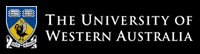School of Computer Science and Software Engineering

# School of Computer Science and Software Engineering

## CITS2200 Data Structures and Algorithms

### Some animals from the complexity zoo

ALL: the class of all languages

Almost-NP: the class of languages that is in NP with probability 1, given a random oracle

BPP: The class of problems for which there is a polynomial algorithm that is correct at least 2/3 of the time.

BQP: The class of problems solveable by a quantum Turing machine in polynomial time, correct at least 2/3 of the time.

Co-NP: The complement of NP

DSPACE(f(n)): The class of problems that may be solved by a Turing machine using O(f(n)) memory.

ELEMENTARY: Problems that can be computed in n-exponential time, for some n

EXP: Problems that can be computed in exponential time

FO: Problems that can be represented in first order logic

L: Problems that may be solved by a Turing machine memory logarithmic in the size of the problem.

NP: The class of dashed hopes and idle dreams (Languages that may be computed by a non-deterministic Turing machine in polynomial time)

P: Problems that may be solved using a deterministic Turing machine in polynomial time

RE: Problems that may be solved using a deterministic Turing machine in a finite amount of time

REG: Regular languages (languages that may be computed using a deterministic finite state machine)

WHILE: A theoretical programming in which all programmes are guarenteed to run in polynomial time.

ZPP: The class of problems that is expected to be solved in polynomial time by a randomized algorithm

In this workshop and the next we will be working through the basics of Java Programming that you would have covered in CITS1001 Java Programming. The following lecture slides cover the material that you should know (also available in note form).
1. Variables

1. What is the difference between an instance variable and a class variable?
How are they accessed?

2. What kind of variable would be used for a constant (such as the weight of an object)?
Where and how are these type of variables declared?

3. What kind of variable would be used for a varying amount (such as the exchange rate from Australian to US dollars)?
Where and how are these type of variables declared?

4. What kind of variable would be used for a loop counter?
Where and how are these type of variables declared?

5. Why should you write:

int[] a;

instead of (as many texts do):

int a[];

2. Methods

1. What is the difference between an instance method and a class method?
How are they accessed?

2. What do the access modifiers `public`, `protected`, (`package`), and `private` mean?

3. What is meant by each part of the statement:

public static void main(String[] args)

4. What kind of "thing" is each part of the statement:

System.out.println(myObject);

How does it work?

3. Logic

Consider the following code blocks:

1. if (!A && !B) instructionBlock1; else instructionBlock2;

2. if (!A || !B) instructionBlock2; else instructionBlock1;

3. if (!A || !B) instructionBlock1; else instructionBlock2;

4. if (!(A || B)) instructionBlock1; else instructionBlock2;

5. if (!(A || B)) instructionBlock2; else instructionBlock1;

6. if (!(A && B)) instructionBlock1; else instructionBlock2;

7. if (!(A && B)) instructionBlock2; else instructionBlock1;

8. Which of them are equivalent?

4. Equals

Consider the following class definition:

public class A { private Object x; private Object y; public A(Object x, Object y) { this.x = x; this.y = y; } public boolean equals(Object o) { if (o == this) return true; else if (o == null) return false; else if (!(o instanceof A)) return false; else { A a = (A)o; return a.x == this.x && a.y == this.y; } } public static void go() { A a1 = new A(new Integer(1), new Integer(2)); A a2 = new A(new Integer(3), new Integer(4)); A a3 = new A(new Integer(1), new Integer(2)); A a4 = a1; System.out.println("a1 == a2 is: " + (a1 == a2)); System.out.println("a1 == a3 is: " + (a1 == a3)); System.out.println("a1 == a4 is: " + (a1 == a4)); System.out.println(); System.out.println("a1 equals a2 is: " + a1.equals(a2)); System.out.println("a1 equals a3 is: " + a1.equals(a3)); System.out.println("a1 equals a4 is: " + a1.equals(a4)); } }

What is printed to the screen when the `go` method is executed, and why?

5. Packages

1. What is a package?

2. What is meant by the statement:

import java.io.*;

6. Exceptions

1. What is the difference between a checked exception and an unchecked exception?

2. What options exist for dealing with exceptions?

7. Interfaces

1. What is the difference between an interface and an abstract class?

2. What differences are there between implementing an interface and extending an abstract class?

# The University of Western Australia

## University information

CRICOS Code: 00126G